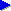Home# Bytes and bytes:How computers (and Java) represent numbers

For many simple programs, it's not too important to know exactly how computers represent numbers. It's often enough to know things in basic terms, such as:

• "a Java int can store a moderately large whole number, positive or negative; it's enough for most loop counters, counting the number of words in a document, storing pixel coordinates etc"
• "a Java long can store a whole number with twice the magnitude of an int; it's more appropriate for larger numbers such as the number of potential bytes in a file, the numbe of milliseconds since a particular point in time etc"

## When do you need to know about how computers represent numbers...?

Sometimes it's useful to have a more precise idea of just how numbers are represented by the computer "under the hood". This can be important in cases such as:

• understanding certain algorithms, such as how to create a hash code or random number generator;
• understanding certain optimisations that are possible, such as using an AND operation instead of a division/modulus, using bit tests to calculate a logarithm, or combining a test for various variables being negative into a single comparison;
• manipulating data, such as when the red/green/blue values of the pixel in an image are combined into a single integer;
• performing data conversion, for example converting data from a system that stores numbers in one format to be compatible with another system that expects them in another format;
• for reducing storage space required by data.

On the next page, we look at binary representation used by computers to store and manipulate numbers. If you're already familiar with binary notation in principle (e.g. because you've studied mathematics), you may prefer to skip straight on to look at how binary digits are grouped into units in computing, starting with bits and bytes.